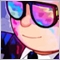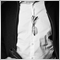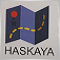# double normalization19

i have a buy order ...that has a stop loss of 1.5 times the ATR (the atr_stoploss_modifier=1.5)

order_num=OrderSend(Symbol(),OP_BUY,SetLotSize(),current_ask,2,current_ask-atr_stoploss_modifier*atr_value ,current_ask+999*_Point*broker_digit_modifier)

^^^^^^^^^^^^^^^^^^^^^^^^^^^^^^^^^

lets say i am trading the EURUSD with a 5 digit broker.

does the stoploass need to have 5 digits exalctly ?

or

if it has more digits (because  when i multiply the atr value with 1.5 it can produce a stop loss with 6 digits)...will it trim it otomaticaly and accept the order?

or

i have to use NormalizeDouble() function1727

You have to use Normalize Double229

Dominik Egert:
You have to use Normalize Double

Agree.2551

jack zorlob:

i have a buy order ...that has a stop loss of 1.5 times the ATR (the atr_stoploss_modifier=1.5)

order_num=OrderSend(Symbol(),OP_BUY,SetLotSize(),current_ask,2,current_ask-atr_stoploss_modifier*atr_value ,current_ask+999*_Point*broker_digit_modifier)

^^^^^^^^^^^^^^^^^^^^^^^^^^^^^^^^^

lets say i am trading the EURUSD with a 5 digit broker.

does the stoploass need to have 5 digits exalctly ?

or

if it has more digits (because  when i multiply the atr value with 1.5 it can produce a stop loss with 6 digits)...will it trim it otomaticaly and accept the order?

or

i have to use NormalizeDouble() function

```  atr_stoploss_modifier=atr_stoploss_modifier/MarketInfo(OrderSymbol(),MODE_POINT); // 1.5 convert Pips
```25060

Dominik Egert: You have to use Normalize Double
Tan Chee Ho: Agree.

Disagree.

NormalizeDouble, It's use is usually wrong,

1. Floating point has infinite number of decimals, it's your not understanding floating point and that some numbers can't be represented exactly. (like 1/10.)
Double-precision floating-point format - Wikipedia, the free encyclopedia

See also The == operand. - MQL4 programming forum 2013.06.07

2. Print out your values to the precision you want with DoubleToString - Conversion Functions - MQL4 Reference.

3. SL/TP (stops) need to be normalized to tick size (not Point) — code fails on metals. (On 5Digit Broker Stops are only allowed to be placed on full pip values. How to find out in mql? - MQL4 programming forum) and abide by the limits Requirements and Limitations in Making Trades - Appendixes - MQL4 Tutorial and that requires understanding floating point equality Can price != price ? - MQL4 programming forum

4. Open price for pending orders need to be adjusted. On Currencies, Point == TickSize, so you will get the same answer, but it won't work on Metals. So do it right: Trailing Bar Entry EA - MQL4 programming forum or Bid/Ask: (No Need) to use NormalizeDouble in OrderSend - MQL4 programming forum

5. Lot size must also be adjusted to a multiple of LotStep and check against min and max. If that is not a power of 1/10 then NormalizeDouble is wrong. Do it right.

6. MathRound() and NormalizeDouble() are rounding in a different way. Make it explicit.
MT4:NormalizeDouble - MQL5 programming forum
How to Normalize - Expert Advisors and Automated Trading - MQL5 programming forum

7. Prices you get from the terminal are already normalized.

8. PIP, Point, or Tick are all different in general.
What is a TICK? - MQL4 programming forum 2014.08.031727

Good hint.... Thank you.

I've come across this many times and I again fell for Normalize Double....